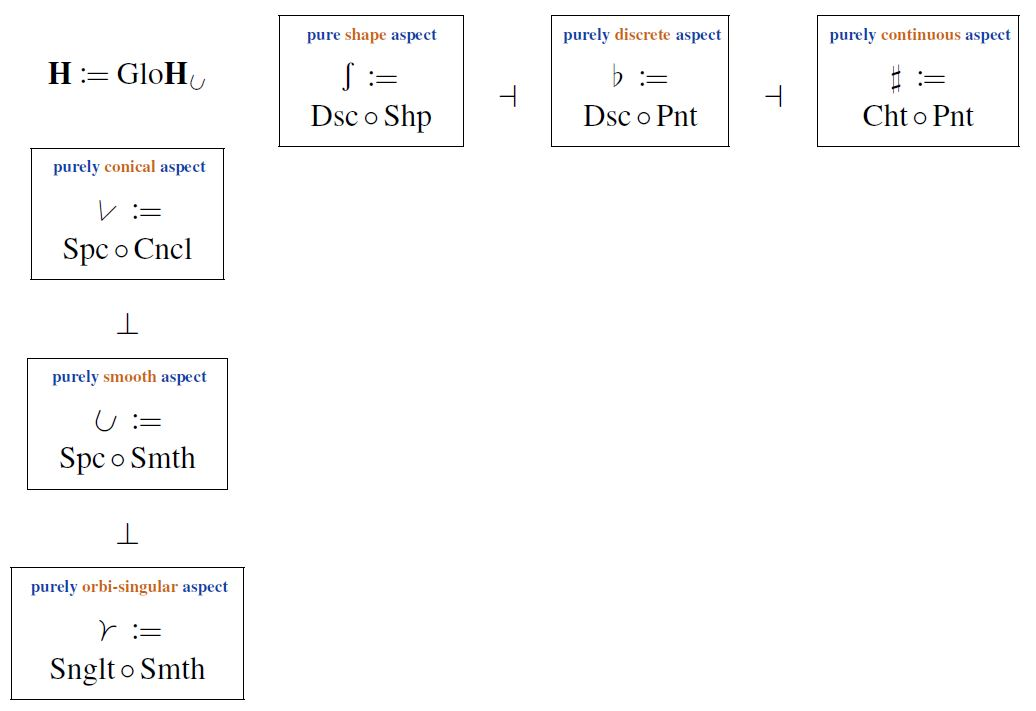# nLab singular cohesion

Contents

### Context

#### Representation theory

representation theory

geometric representation theory

# Contents

## Idea

What is called singular cohesion in SaSc 2020, following observations in Rezk 2014, is a form of cohesion on $\infty$-toposes which enhances any base notion of “smooth” cohesive geometry to a “singular-smooth” cohesive geometry which reflects the possible presence of orbi-singularities.

For example, where smooth manifolds are faithfully embedded into the cohesive $\infty$-topos of smooth $\infty$-groupoids, so their orbifolds are faithfully embedded among singular-smooth $\infty$-groupoids such that, for global quotient orbifolds, their cohesive shape is the homotopy type of the underlying G-spaces in proper equivariant homotopy theory.

For the base $\infty$-topos $Grpd_\infty$, embodying plain homotopy theory, its singular-cohesive enhancement is global equivariant homotopy theory and hence every singular-cohesive $\infty$-topos is cohesive over (in particular) global equivariant homotopy theory, hence may be thought of as embodying a “globally equivariant and cohesive” homotopy theory.

In fact, every slice of a singular-cohesive $\infty$-topos over the canonical $G$-orbi-singularity $\boxed{\prec}\mathbf{B}G$ is cohesive over the $G$-equivariant homotopy theory over the given smooth cohesive base, with the inverse image of the cohesive geometric morphism being the embedding of cohesive $G$-orbispaces.

### Globally equivariant geometric homotopy theory

(1)$\array{ Singularities &\coloneqq& Grpd^{fin}_{1, \geq 1} &\xhookrightarrow{\;}& Grp_\infty \\ \boxed{\prec}\mathbf{B}G & \mapsto & B G }$

(sometimes called the “global orbit category”), hence the full sub (2,1)-category of groupoids on the delooping groupoids of finite groups $G$.

Then for $\mathbf{H}_{\subset}$ any base $\infty$-topos, the $\infty$-category of $\infty$-presheaves over $Singularities$ (1)

$\mathbf{H} \,\coloneqq\, Glo \mathbf{H}_{\subset} \;\coloneqq\; PSh_\infty \big( Singularities \,, \mathbf{H}_{\subset} \big) \,,$

which may be called the globally equivariant homotopy theory over the geometric homotopy theory $\mathbf{H}_{\subset}$, is cohesive over $\mathbf{H}_{\subset}$ (as is any $\infty$-presheaf $\infty$-topos over an $\infty$-site with a terminal object, see e.g. at adjoint quadruple the section Cohesion).

In fact, for any $\boxed{\prec}\mathbf{B}G \,\in\, Singularities$, the slice $\infty$-topos $Glo(\mathbf{H}_{\subset})_{/\boxed{\prec}\mathbf{B}G}$ is cohesive over the $G$-equivariant homotopy theory, hence over the $\infty$-topos of $\infty$-presheaves over the actual orbit category of $G$:

$G \mathbf{H}_{\subset} \;\coloneqq\; Psh_\infty \big( G Orbits ,\, \mathbf{H}_{\subset} \big) \,.$

For the discrete equivariance groups considered here, this follows on general abstract grounds (see at Adjoint quadruple – Cohesion) from the full sub (2,1)-category inclusion

$\Singularities_{/\boxed{\prec}\mathbf{B}G} \underoverset {\hookleftarrow} {\overset{\tau_0}{\longrightarrow}} {\;\;\; \bot \;\;\;} G Orbits \,,$

as discussed at cohesion of global- over G-equivariant homotopy theory (highlighted in Rezk 2014, Sec. 7).

### Globally equivariant cohesive homotopy theory

This means that if $\mathbf{H}_{\subset}$ is itself cohesive over $Grpd_\infty$ then $\mathbf{H} = Glo(\mathbf{H}_{\subset})$ carries the following system of adjoint quadruples:

### Singular cohesion

From this is obtained, in particular, a pair of adjoint triples of adjoint modalities on $\mathbf{H} \,\coloneqq\, Glo(\mathbf{H}_{\subset})$ which jointly reflect that its objects are geometric homotopy types whose geometric nature is “cohesive with orbi-singularities”:### Orbifolds among singular-smooth $\infty$-groupoids

For example, when $\mathbf{H}_{\subset} \,=\,$ $SmthGrpd_\infty$, containing diffeological spaces as the full sub-$\infty$-category of 0-truncated concrete objects

$DiffSpc \xhookrightarrow{ i_{\tau_0, \sharp_1} } SmthGrpd_\infty \,,$

then the singular-cohesive $\infty$-topos $\mathbf{H} \,\coloneqq\, Glo(SmthGrpd_\infty)$ of singular-smooth $\infty$-groupoids contains diffeological orbifolds as a full sub (2,1)-category of orbi-singular-, hence of $\boxed{\prec}$-modal objects

$\array{ DiffOrbfld &\xhookrightarrow{\;}& LieGrpd &\xhookrightarrow{ \;}& Glo(SmthGrpd_\infty) \\ && X \sslash G &\mapsto& \boxed{\prec}(X \sslash G) \mathrlap{\,,} }$

and in such a way that their shape

$\esh \, \prec \, (X \!\sslash\! G) \;\;\in\;\; G Grpd_\infty \xhookrightarrow{ Dsc } (G SmthGrpd_\infty)_{/\boxed{\prec}\mathbf{B}G} \xhookrightarrow{ G Spc } \mathbf{H}_{/\boxed{\prec}\mathbf{B}G}$

is the incarnation of the D-topological space underlying $X$ as a G-space in $G$-equivariant homotopy theory (via Elmendorf's theorem).

The cohesion of global- over G-equivariant homotopy theory was first observed in

with emphasis on characterization of the full image under $G Spc$ of orbispaces in sliced global homotopy theory.

The above combination with smooth cohesion to singular cohesion was considered in

in discussion of orbifold cohomology, where the above notation is taken from, and in

in discussion of equivariant principal $\infty$-bundles, where the above diagrams are taken from.